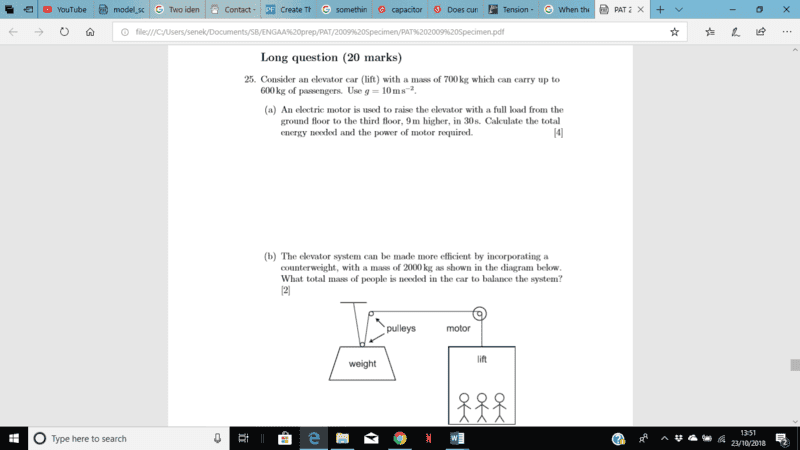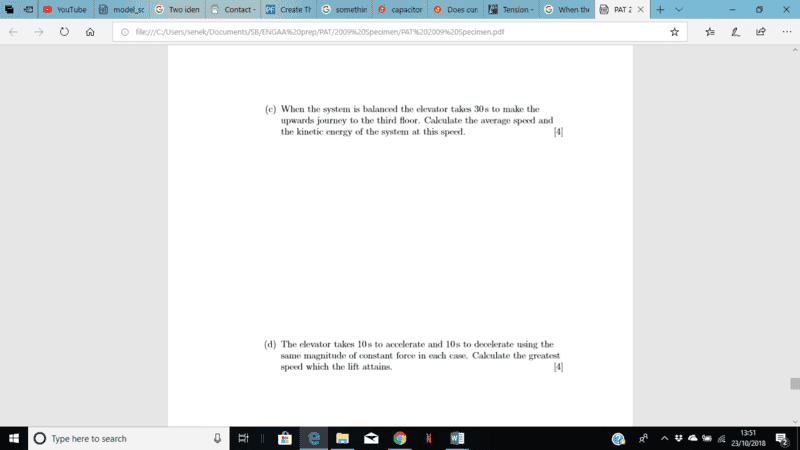# Pulley system Part c

## Homework Statement2. Relevant equation

## The Attempt at a Solution

...[/B]
Part c)
I'm confused as to how the actual pulley system works. If its balanced wouldn't it be stationary. How does it move?
I thought that since its balanced the acceleration of the system is constant and therefore to find the average kinetic energy of the system, I would just do 1/2 x (2000 + 1000) x (9/30)^2
The answers say that the weight only moves half as far. How do you know this?

#### Attachments

•